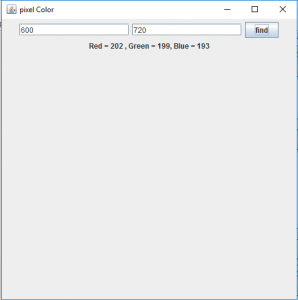Open in App
Not now

# Java Robot Class | Get the pixel Color of a given point

• Difficulty Level : Medium
• Last Updated : 14 Apr, 2021

Robot is part of java.awt package . Robot class is basically used to generate native system input events for the purposes of self- running demos, test automation, and other application where control over mouse and keyboard is used.
Robot class generates events that can be used to control mouse, keyboard and can be used to take screenshot of the screen.
In this article we will discuss how to get the pixel color of the point on the screen mentioned by the user.
Method used :

```getPixelColor(int x, int y)
This function returns an object of the color class
of the given screen coordinates. ```

In the following program we will print a label that will contain the RGB values of the pixel entered and the text of the label will be of the pixel Color

## Java

 `// Java program to get the pixel color of``// given screen coordinates``import` `java.awt.*;``import` `javax.swing.*;``import` `java.awt.event.*;``public` `class` `color ``extends` `JFrame``    ``implements` `ActionListener {``    ``// textfield to get x, y coordinates``    ``static` `JTextField x, y;` `    ``// button``    ``static` `JButton b;` `    ``// create a frame``    ``static` `JFrame f;` `    ``// label``    ``static` `JLabel l;` `    ``public` `static` `void` `main()``    ``{` `        ``// create a frame``        ``f = ``new` `JFrame(``"pixel Color"``);` `        ``// label to show the RGB value``        ``l = ``new` `JLabel(``"no value"``);` `        ``// create the text field``        ``x = ``new` `JTextField(``16``);``        ``y = ``new` `JTextField(``16``);` `        ``// create a button``        ``b = ``new` `JButton(``"find"``);` `        ``// create an object of the class``        ``color co = ``new` `color();` `        ``// add ActionListener``        ``b.addActionListener(co);` `        ``// create a panel``        ``JPanel p = ``new` `JPanel();` `        ``// add textfield and button to the panel``        ``p.add(x);``        ``p.add(y);``        ``p.add(b);``        ``p.add(l);` `        ``// add the panel``        ``f.add(p);` `        ``// set the size of the frame``        ``f.setSize(``500``, ``500``);``        ``f.show();``    ``}` `    ``// if the button is pressed``    ``public` `void` `actionPerformed(ActionEvent e)``    ``{``        ``String s = e.getActionCommand();``        ``if` `(s.equals(``"find"``)) {``            ``int` `xp, yp;` `            ``// get user inputs of x and y position``            ``xp = Integer.parseInt(x.getText());``            ``yp = Integer.parseInt(y.getText());` `            ``// try and catch block to handle exceptions``            ``try` `{``                ``// create an object of robot class``                ``Robot r = ``new` `Robot();` `                ``// get the pixel color``                ``c = r.getPixelColor(xp, yp);``            ``}``            ``catch` `(Exception evt) {``                ``// print error message``                ``System.err.println(evt.getMessage());``            ``}` `            ``Color c;` `            ``// set the RGB value to the label``            ``// and to its foreground``            ``l.setForeground(c);``            ``l.setText(``"Red = "` `+ c.getRed() + ",``Green = ``" + c.getGreen() + "``, Blue = " + c.getBlue());``        ``}``    ``}``}`

Output :Note : the following program might not run in an online compiler please use an offline IDE.

My Personal Notes arrow_drop_up